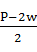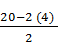### Sample Problem

Solve this perimeter equation for q in terms of P and w: P = 2w + 2q

What is the value of q when w = 4 and P = 20?

#### Solution

P = 2w + 2q

P – 2w = 2q

q =When w = 4 and P = 20,

q =q == 6